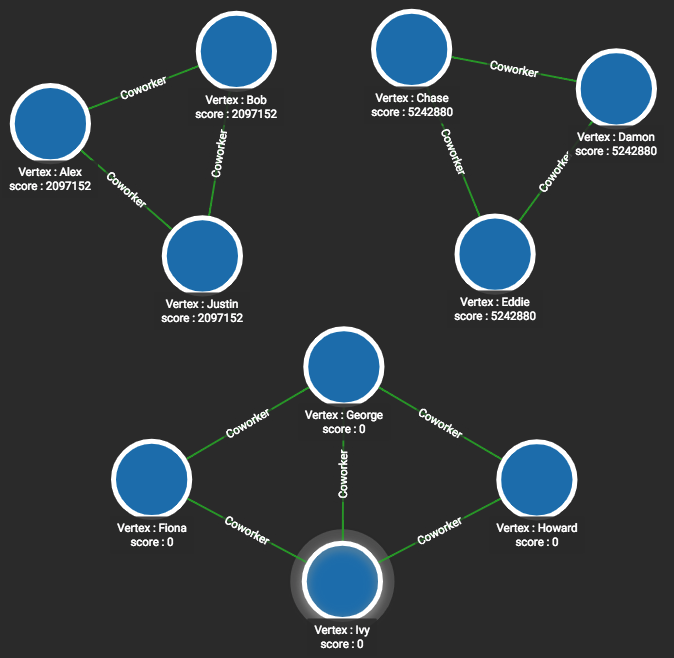# Weakly Connected Components

A component is the maximal set of vertices, plus their connecting edges, which are interconnected. That is, you can reach each vertex from each other vertex. In the example figure below, there are three components.

This particular algorithm deals with undirected edges. If the same definition (each vertex can reach each other vertex) is applied to directed edges, then the components are called Strongly Connected Components. If you have directed edges but ignore the direction (permitting traversal in either direction), then the algorithm finds Weakly Connected Components.

## Specifications

``````tg_conn_comp (SET<STRING> v_type, SET<STRING> e_type, INT output_limit = 100,
BOOL print_accum = TRUE, STRING result_attr = "", STRING file_path = "")``````
Characteristic Value

Result

Assigns a component id (INT) to each vertex, such that members of the same component have the same id value.

Input Parameters

• `SET<STRING> v_type`: Names of vertex types to use

• `SET<STRING> e_type`: Names of edge types to use

• `INT output_limit`: If >=0, max number of vertices to output to JSON.

• `BOOL print_accum`: If True, output JSON to standard output

• `STRING result_attr`: If not empty, store community ID values (INT) to this attribute

• `STRING file_path`: If not empty, write output to this file.

Result Size

V = number of vertices

Time Complexity

O(E*d), E = number of edges, d = max(diameter of components)

Graph Types

Undirected edges

## Example

It is easy to see in this small graph that the algorithm correctly groups the vertices:

• Alex, Bob, and Justin all have Community ID = 2097152

• Chase, Damon, and Eddie all have Community ID = 5242880

• Fiona, George, Howard, and Ivy all have Community ID = 0

Our algorithm uses the TigerGraph engine’s internal vertex ID numbers; they cannot be predicted.

``RUN QUERY tg_conn_comp(["Person"], ["Coworker"], _, _, _, _)``